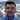# Solution to JavaScript's 0.1 + 0.2 = 0.30000000000000004 problem?

January 08, 2015

Open your JavaScript console and try this:

``````> 0.1 + 0.2 == 0.3

false

> 0.1 + 0.2

0.30000000000000004``````

You may ask yourself Is floating point math broken?.  The answer is that JavaScript uses floating point math based on the IEEE 754 standard, the same as Java’s `double`. If you want the details on what that means, either quickly read The Floating-Point Guide - What Every Programmer Should Know About Floating-Point Arithmetic or fully digest What Every Computer Scientist Should Know About Floating-Point Arithmetic.

But if I really want `0.1 + 0.2 == 0.3` then what do I do?  The Java BigDecimal class solved this problem, and there similar solutions for JavaScript: GWT compiled to JavaScript version; a JavaScript translation of the ICU4J’s `com.ibm.icu.math.BigDecimal`.

There are also other JavaScript libraries like big.js.  Here is big.js in action from the JavaScript console:

``````> Big(0.1).plus(0.2).eq(0.3)

true

> Big(0.1).plus(0.2).toString()

"0.3"``````

How have other teams solved this problem?  Is there a de facto JavaScript library to use that has been well tested?  Would a common library be useful?Software Architect. Web Platform. JavaScript. Cloud. Information Security. Speaker.